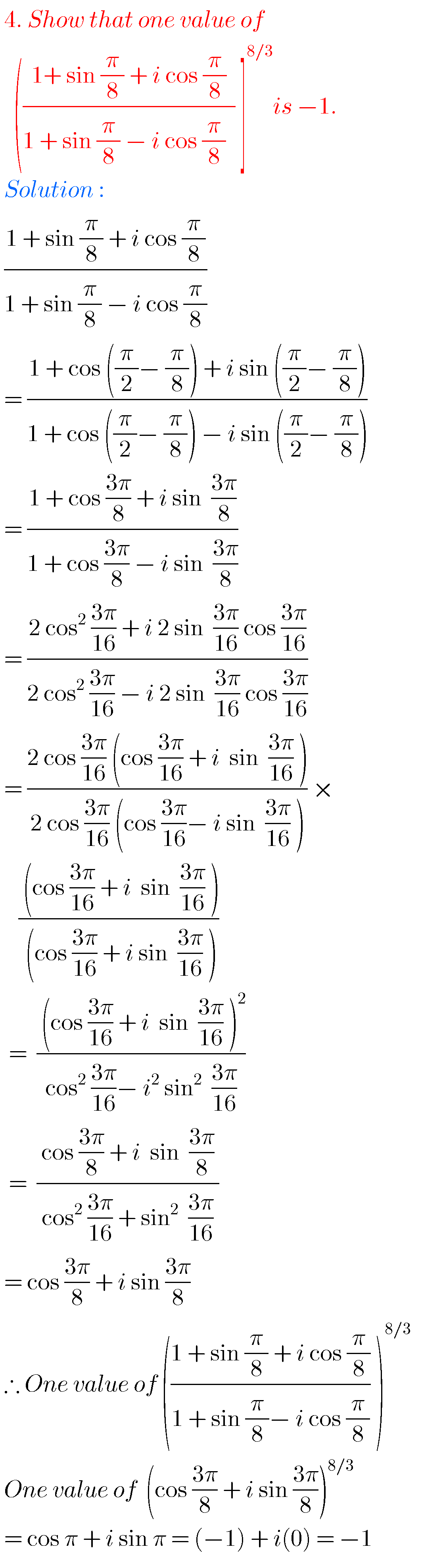## Intermediate maths solutions for IIA

Inter maths solutions for IIA Intermediate maths 2a textbook exercise solutions for all chapters are given. These are very easy to understand. First you study the textbook lessons very well. Then observe the example problems and solutions and try them. Inter second year maths IIA solutions are given below. Observe the solutions and try them …